# Exploratory data analysis for geostatistics: Voronoi diagrams

Following the article   Introduction to exploratory data analysis for geostatistics   we will discuss all the available tools to carry out the exploratory analysis of spatialized data. We have discussed   the histograms ,    the QQ-Plots , and now we will address the Voronoi maps.

We must introduce a notion not yet introduced in the previous articles that concerns the extent or influence of a phenomenon. In geostatistics we can consider two types of extension for a phenomenon: GLOBAL or LOCAL extent.

Global and local phenomena.

We refer to a global phenomenon when we use as reference all the available data. We refer to a local phenomenon when we use as reference a sampling point and its neighbouring points.

A simple example is when we refer to outliers. If we look for global outliers, we will look for values ​​that are outside the logical range of our data. For example, if we have a batch of seawater temperature data, with values ​​that are between 2 ° C and 16 ° C, a value of -3 ° C or a value of 35 ° C, will appear as   global aberrant values. Suppose that our measurements are spread over a whole year and that we have in winter the following series of values ​​2 ° C, 2.5 ° C, 11 ° C, 2.2 ° C, 2.5 ° C. The value 11 ° C is not aberrant from a global point of view, because in the course of the year it can appear quite often. But since it is found in the middle of much lower and regular temperatures we can deduce that it is a local aberrant value.

The histograms and the QQ-plot that we have already discussed are global tools. They allow us to work and understand phenomena that affect all our data. With the Voronoi maps we will tackle tools that will allow us to   visualize and understand local phenomena, ie they will only concern a part of our data.

The Voronoi maps

The Voronoi maps are built from a series of polygons formed around the location of each sampling point.

The Voronoi polygons are created so that each location in a polygon is closer to the sampling point present in that polygon rather than to any other sampling point.For example, in this figure, the yellow dot is surrounded by a polygon, displayed in red. Each location in the red polygon is closer to the yellow sampling point than to any other sampling point (dark blue dots).

After creating the polygons, the   neighbours of a sampling point are defined as any other sampling point whose polygon shares a border with the chosen sampling point. The blue polygons all share a border with the red polygon, so the sampling points in the blue polygons are   neighbours of the clear green sampling point.

Using this neighbourhood definition, an array of local statistics can be calculated. For example, a local average will be calculated by using the average of the sampling points in the central polygon and the neighbouring polygons (red and blue polygons). This average will then be assigned to the red polygon. After repeating this task for all the polygons and their neighbours, a colour scale will show the relative values ​​of the local averages, which allows to visualize regions of high and low values.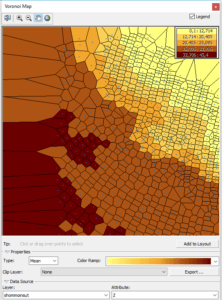At the top right, the colour scale indicates the values ​​of the calculated averages. We can see that the top and right corner have the lowest values ​​of the set and the bottom and left corner have the highest values.

The different Geostatistical Analyst Voronoi maps

The tool Geostatistical Analyst Voronoi map provides a number of methods for assigning or calculating values ​​to the polygons.

Let’s first look at the list of possibilities and how they are calculated. Then we will then discuss their use.

MAPS TYPES

Simple : The value assigned to each polygon a cell is the value of the sampling point of that polygon.

Average : The value assigned to a polygon is the average calculated from this polygon and its neighbours.

Mode : All polygons are classified in   five class intervals. The value assigned to a polygon is the most current value (mode) between the polygon and its neighbours.

Cluster : All polygons are classified into five colour class intervals. If the class range of the polygon is different from all its neighbours, the cell is grey (to distinguish it from its neighbours).

Entropy : All polygons are classified into five classes using the   smart quartiles method, a variation of the quartile method. Entropy is calculated using the following formula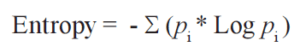Where pi is the proportion of polygons, among the central polygon and the neighbouring polygons, for each of the five classes, and Log is the logarithm base 2.

Since this is not simple, let’s look at an example. We have a polygon with 5 neighbouring  polygons. We apply the smart quartiles method and we obtain 3 class 1 polygons, 1 class 3 polygon and 2 class 5 polygons.We will have an entropy

– [0.6 *   -0.736966   + 0.2 * -2.321928   + 0.4 *   -1.321928] = 1.4353

In all cases we will have values ​​ranging from 0 to 2.322.

If all polygons (central polygon and neighbours) have the same class, the entropy is zero (1 * log2 (1)).

If we find the five classes, each one will have a proportion 0.2 and the resulting entropy will be 2.322.

Median: The value assigned to a cell is the median value calculated from the frequency distribution of the cell and its neighbours.

Standard Deviation: The value assigned to a cell is the standard deviation calculated from the cell and its neighbours.

Interquartile Deviation: The first and third quartiles are calculated using the frequency distribution of a polygon and its neighbours.
The value assigned to the cell is calculated by subtracting the first quartile value from the third quartile value:

• the 1st quartile is the data in the series that separates the bottom 25% of the data;
• the 2nd quartile is the data of the series that separates the series into two parts (50%) of the series;
• the 3rd quartile is the data in the series that separates the top 25% of the data.

The difference between the third quartile and the first quartile is called the interquartile deviation; it is a dispersion criterion of the series. Dispersion represents the variability or range of different values ​​a variable can take. The interquartile deviation is the range of the statistical series after elimination of 25% of the lowest values ​​and 25% of the highest values. This measure is more robust than the extent (range =   ), which is sensitive to extreme values.

USAGE OF DIFFERENT MAP TYPES

The different Voronoi statistics are used for different purposes.
Statistics can be grouped into the following general functional categories:

Local smoothing tools:

• average map
• mode map
• median map

By calculating one of the three statistical variables for each point and its neighbours, the variation between each polygon and its neighbours becomes less abrupt. We get a smoother map of our data. This is useful when there is too much variation between neighbouring  points which results in the global map masking or making it more difficult to observe the global phenomena.The map on the left shows the simple Voronoi polygons, ie each point is represented by its real value. The map on the right shows the average Voronoi polygons. Each polygon has as value equal to the average of its value and its neighbours. The background noise from the centre of the map is “smoothed”  thanks to the use of the average.

Tools for visualizing local variability

• standard deviations map
• interquartile deviation map
• entropy map

If the smoothing tools (average, mode, median) are tools that refer to what we can call the central tendency of a distribution, these three tools refer to the dispersion of the distributions.

If we observe a lot of difference between the neighbouring values, we will state that the values ​​have a strong dispersion and variability.

On the other hand, the notion of   “very much”   is a relative notion. Let’s look the standard deviations map: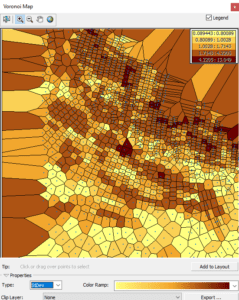The colour scale is always the same, whatever the dispersion of the values. To fully interpret this image, you must know which data is involved and whether a maximum variability   from 4 to 13 is logical or not.

On the other hand, what we can immediately understand is that the light areas are the areas where the relative variability is low and the darker areas those where the variability is very strong.

Take the interquartile deviation map:This map is another measure of dispersion. We use the values ​​of the point and its neighbours, eliminate the 25% lower and higher, and display the min and max values ​​of the remaining points.

In short, we eliminate the extreme values ​​and display a range of variation. Dark areas vary between 4 and 10.

Since we, now, know that these data correspond to depths, we can deduce that the dark areas of the two previous maps correspond to the zones of steeper slope, ie where the values ​​change faster. The light areas correspond to rather flat areas.

The interpretation of these two types of maps depends on the knowledge of the data, because the variability will always be expressed in five classes, with different boundaries depending on the data. On the other hand, the entropy map does not look alike. It always has 5 classes but class boundaries do not depend on the processed data. They are fixed.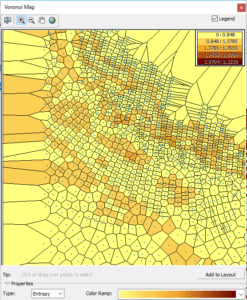If all the polygons (nearest neighbours) look alike, the value of the entropy will be 0. If all the polygons are different, the value is 2.32.

As its name suggests, the entropy map is a measure of   “disorder”. If we find areas with high entropy (this is not the case in our example map) a detour to try to understand the reasons is required.

Search for outliers

It is important to identify outliers for two reasons: they may be actual anomalies of the phenomenon, or the value may have been wrongly measured or recorded.
If an aberration is a real anomaly in the phenomenon, it is perhaps the most important point to study and to understand the phenomenon. For example, a sample on the vein of an ore could appear as an outlier, and it is precisely this location that is the most important goal for a mining company.
If outliers are caused by errors in data entry or by any other clearly incorrect reason, they must be corrected or deleted before creating a surface. Outliers can have several detrimental effects on your interpolated surface, with effects on semi-variogram modelling and its influence on neighbouring values.

Voronoi maps created using cluster and entropy methods can be used to help identify possible outliers.
Entropy values ​​provide a measure of dissimilarity between neighbouring polygons. In nature, you expect that things close together are more   similar than things further away. Therefore, local outliers can be identified by high entropy areas.

The cluster method identifies polygons that are dissimilar to their surrounding neighbours. You expect the value stored in a particular polygon to be similar to, at least, one of its neighbours. Therefore, this tool can be used to identify possible local outliers: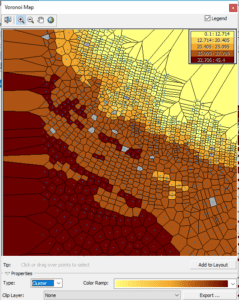The cluster includes all the points and classifies these values ​​into five classes. For each polygon we display its class, if and only if, at least one neighbouring polygon belongs to the same class. If all neighbouring polygons belong to different classes, the polygon is displayed in grey.

On the previous image, it will be useful to click on each grey polygon and observe its value on the map of points: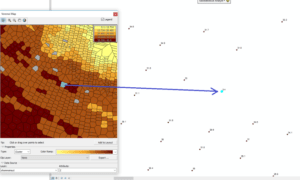In this example, a value of 3.4 is observed among values ​​of the order of 30. It may be a decimal entry error.

In the next article we will see how to analyse the data trends.